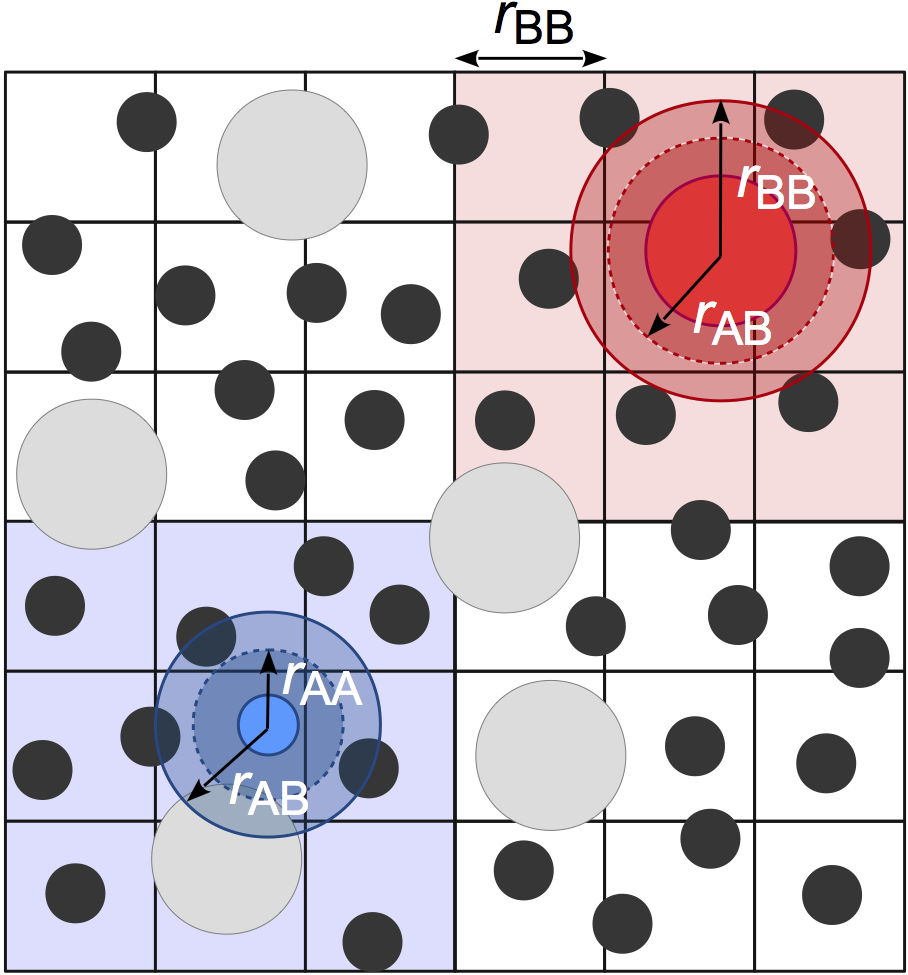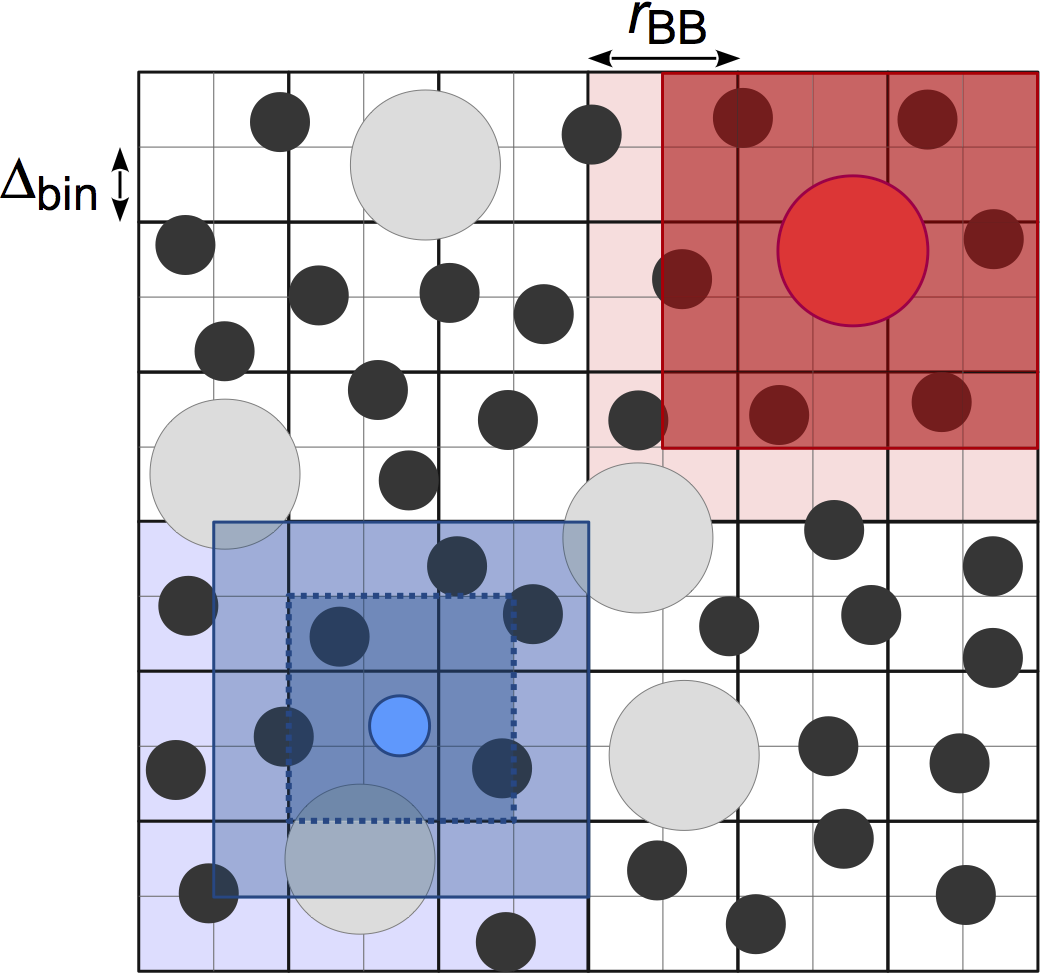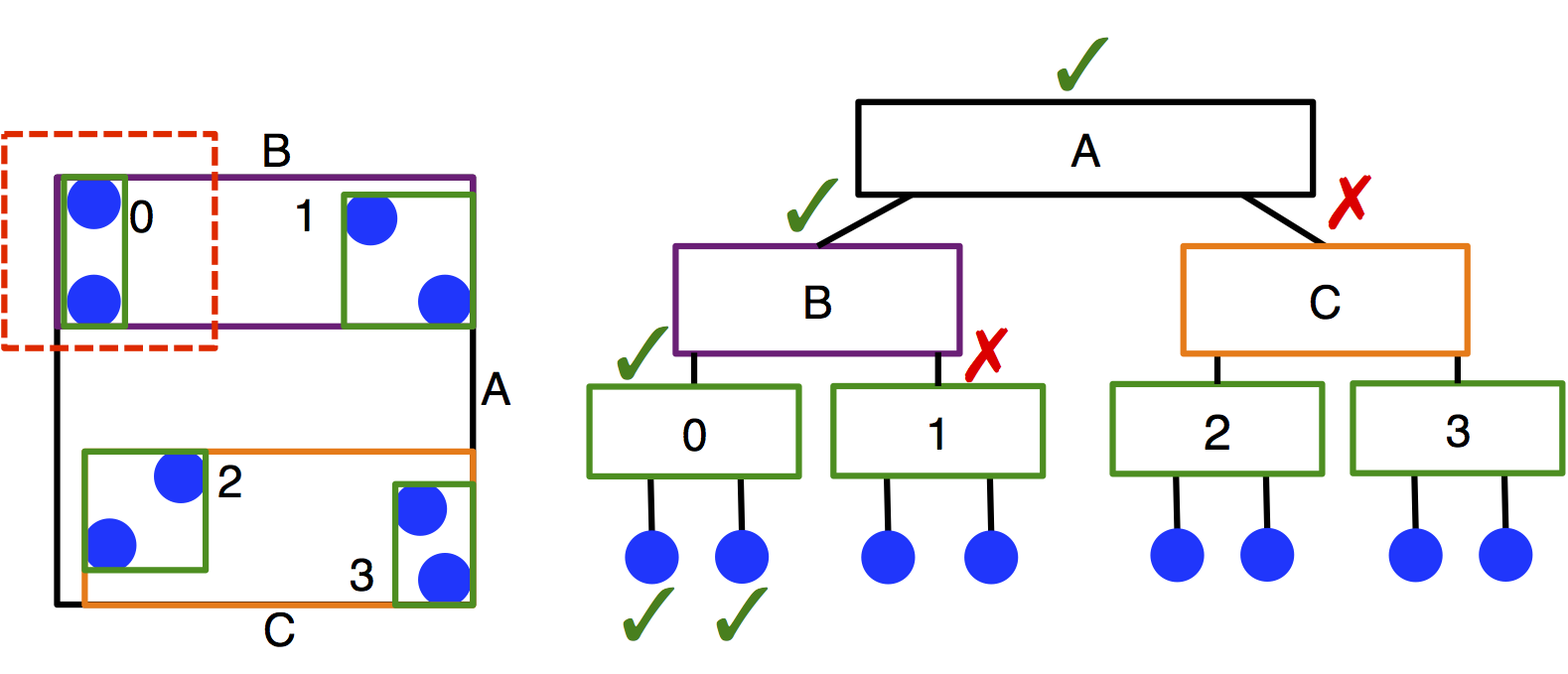# Neighbor lists¶

## Overview¶

Neighbor lists accelerate the search for pairs of atoms that are within a certain cutoff radius of each other. They are most commonly used in hoomd.md.pair to accelerate the calculation of pair forces between atoms. This significantly reduces the number of pairwise distances that are evaluated, which is $$O(N^2)$$ if all possible pairs are checked. A small buffer radius (skin layer) r_buff is typically added to the cutoff radius so that the neighbor list can be computed less frequently. The neighbor list must only be rebuilt any time a particle diffuses r_buff/2. However, increasing r_buff also increases the number of particles that are included in the neighbor list, which slows down the pair force evaluation. A balance can be obtained between the two by optimizing r_buff.

A simple neighbor list is built by checking all possible pairs of atoms periodically, which makes the overall algorithm $$O(N^2)$$. The neighbor list can be computed more efficiently using an acceleration structure which further reduces the complexity of the problem. There are three accelerators implemented in HOOMD-blue:

More details for each can be found below and in M.P. Howard et al. 2016 and M.P. Howard et al. 2019 . Each neighbor list style has its own advantages and disadvantages that the user should consider on a case-by-case basis.

## Cell list¶

The cell-list neighbor list (hoomd.md.nlist.cell) spatially sorts particles into bins that are sized by the largest cutoff radius of all pair potentials attached to the neighbor list. For example, in the figure below, there are small A particles and large B particles. The bin size is based on the cutoff radius of the largest particles $$r_{\rm BB}$$. To find neighbors, each particle searches the 27 cells that are adjacent to its cell, which are shaded around each particle. Binning particles is O(N), and so neighbor search from the cell list is also O(N).This method is very efficient for systems with nearly monodisperse cutoffs, but performance degrades for large cutoff radius asymmetries due to the significantly increased number of particles per cell and increased search volume. For example, the small A particles, who have a majority of neighbors who are also A particles within cutoff $$r_{\rm AA}$$ must now search through the full volume defined by $$r_{\rm BB}$$. In practice, we have found that this neighbor list style is the best option for most users when the asymmetry between the largest and smallest cutoff radius is less than 2:1, but the performance of hoomd.md.nlist.tree may still be competitive at smaller asymmetries.

Note

Users may find that the cell-list neighbor list consumes a significant amount of memory, especially on CUDA devices. One cause of this can be non-uniform density distributions because the memory allocated for the cell list is proportional the maximum number of particles in any cell. Another common cause is large system volumes combined with small cutoffs, which results in a very large number of cells in the system. In these cases, consider using hoomd.md.nlist.stencil or hoomd.md.nlist.tree.

## Stenciled cell list¶

Performance of the simple cell-list can be improved in the size asymmetric case by basing the bin size of the cell list on the smallest cutoff radius of all pair potentials attached to the neighbor list (P.J. in’t Veld et al. 2008). From the previous example, the bin size is now based on $$r_{\rm AA}$$. A stencil is then constructed on a per-pair basis that defines the bins to search. Some particles can now be excluded without distance check if they lie in bins outside the stencil. The small A particles only need to distance check other A particles in the dark blue cells (dashed outline). This reduces both the number of distances evaluations and the amount of particle data that is read.We have found that the stenciled cell list (hoomd.md.nlist.stencil) is most useful when a cell list performs well, but is too memory intensive. It may also be useful for some systems with modest size asymmetry or many types. The memory consumed by the stenciled cell list is typically much lower than that used for a comparable simple cell list because of the way the stencils constructed to query the cell list. However, this comes at the expense of higher register usage on CUDA devices, which may lead to reduced performance compared to the simple cell list in some cases depending on your CUDA device’s architecture.

Note

Users may still find that the stenciled cell list consumes a significant amount of memory for systems with large volumes and small cutoffs. In this case, the bin size should be made larger (possibly at the expense of performance), or hoomd.md.nlist.tree should be used instead.

## LBVH tree¶

Linear bounding volume hierarchies (LBVHs) are an entirely different approach to accelerating the neighbor search. LBVHs are binary tree structures that partition the system based on objects rather than space (see schematic below). This means that the memory they require scales with the number of particles in the system rather than the system volume, which may be particularly advantageous for large, sparse systems. Because of their lightweight memory footprint, LBVHs can also be constructed per-type, and this makes searching the trees very efficient in size asymmetric systems. The LBVH algorithm is O(N log N) to search the tree.We have found that LBVHs (hoomd.md.nlist.tree) are very useful for systems with size asymmetry greater than 2:1 between the largest and smallest cutoffs. These conditions are typical of many colloidal systems. Additionally, LBVHs can be used advantageously in sparse systems or systems with large volumes, where they have less overhead and memory demands than cell lists. The performance of the LBVHs has been improved since their original implementation (see M.P. Howard et al. 2019 for details), making them more competitive for different types of systems.

## Multiple neighbor lists¶

Multiple neighbor lists can be created to accelerate simulations where there is significant disparity in the pairwise cutoffs between pair potentials. If one pair force has a maximum cutoff radius much smaller than another pair force, the pair force calculation for the short cutoff will be slowed down considerably because many particles in the neighbor list will have to be read and skipped because they lie outside the shorter cutoff. Attaching each potential to a different neighbor list may improve performance of the pair force calculation at the expense of duplicate computation of the neighbor list. When using multiple neighbor lists, it may be advantageous to adopt two different neighbor list styles. For example, in a colloidal suspension of a small number of large colloids dispersed in many solvent particles, a modest performance gain may be achieved by computing the solvent-solvent neighbors using hoomd.md.nlist.cell, but the solvent-colloid and colloid-colloid interactions using hoomd.md.nlist.tree. Particles can be excluded from neighbor lists by setting their cutoff radius to False or a negative value.